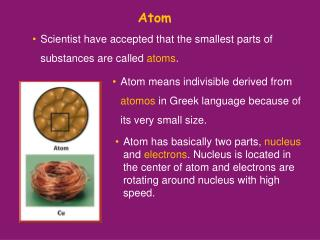Download PresentationAtom

# Atom - PowerPoint PPT PresentationDownload Presentation## Atom

- - - - - - - - - - - - - - - - - - - - - - - - - - - E N D - - - - - - - - - - - - - - - - - - - - - - - - - - -
##### Presentation Transcript

1. Atom • Scientist have accepted that the smallest parts of substances are called atoms. • Atom means indivisible derived from atomos in Greek language because of its very small size. • Atom has basically two parts, nucleus and electrons. Nucleus is located in the center of atom and electrons are rotating around nucleus with high speed.

2. Subatomic Particles • Scientist believed that atoms were indivisible up to 20th century. Today it is well known that atoms have subatomic particles, called protons, neutrons and electrons. • Protons are positively charged particles found in the nucleus of an atom, and denoted by “p”. Each element has certain number of protons which differ the element from others.

3. Subatomic Particles • Neutrons are neutral particles found in the nucleus of an atom, and denoted by “n”. • Electrons are negatively charged particles placed around the nucleus of an atom, and shown by “e-”. • Protons and neutrons almost have the same masses, but electrons have negligible mass with respect to protons and neutrons.

4. Subatomic Particles

5. Subatomic Particles

6. Subatomic Particles

7. Subatomic Particles • Neutral atoms have the equal number of protons and electrons. • Electrons are rotating in certain places called orbit,energy level or shell. Energy levels are represented by letters, K, L, M, N, O…etc, or numbers, 1, 2, 3, 4, …etc. • Each shell can hold a certain number of electrons calculated by the equation of “2n2” where n refers to number of shell. • The electrons located in the outermost shell of atoms are called valence electrons.

8. Subatomic Particles

9. Subatomic Particles • In the 1st shell, No. of e- = 2x12 = 2e- • In the 2nd shell, No. of e- = 2x22 = 8e- • In the 3rd shell, No. of e- = 2x32 = 18e- • In the 4th shell, No. of e- = 2x42 = 32e- Example 1 Show the electron configuration of 6C and 13Al atoms.

10. Subatomic Particles

11. Isotopes • Isotope atoms have the same number of protons but different number of neutrons. Example 2 atoms are isotopes. All they have 6 protons but 6, 7 and 8 neutrons respectively. • They have similar chemical properties but different physical properties.

12. Isotopes

13. Isotones • Isotones, atoms with the same number of neutrons, but different numbers of protons. Example 3 Example 3 Example 3 Example 3 atoms are isotones. atoms are isotones. atoms are isotones. atoms are isotones. Each have 16 neutrons but 15 and 16 protons respectively. • Isotone atoms are completely different atoms, they have different chemical and physical characteristics.

14. Ions • Electrically charged atoms are called ions. • When an atom loses electrons it becomes positively charged ion, called cation. • When an atom gains electrons it becomes negatively charged ion, called anion. • Charge of an atom, q , can be found with q = p - e.

15. Ions

16. Ions Example Find the charge and ion type of atom.

17. Ions Solution

18. The Atomic Terminology 1. Atomic Number, Z • Atomic number = Number of Protons • Each type of atom has different number of protons. • For a neutral atom, • Atomic number = Number of protons = Number of electrons • Z = p = e

19. 2. Atomic Mass Number, A • Atomic mass number = Number of Protons + Number of Neutrons • A = p + n Example Fill in the blanks in the table below.

20. Solution

21. Example What is the number of protons and atomic mass number of Zn.

22. Solution A = 30 + 35 A = 65 +2 = p – 28 p = 30

23. Example Cr+3 ion has 21 electrons and its atomic mass number is 52. What is the number of neutrons for Cr ?

24. Average Atomic Mass • Most of the elements in nature are found as a mixture of isotope atoms. • The average atomic mass is the average masses of natural isotopes of an element. Average Atomic Mass = A1x% of 1st isotope + A2x% of 2nd isotope + …… A1 and A2 are atomic mass numbers of natural isotopes.

25. Example Naturally occurring Ga consists of 60% 69Ga and 40% 71Ga. What is the average mass of Ga? Solution Average Atomic Mass = A1x% of 1st isotope + A2x% of 2nd isotope + …… = 69.80 amu

26. Example Find the average atomic mass of Pb?